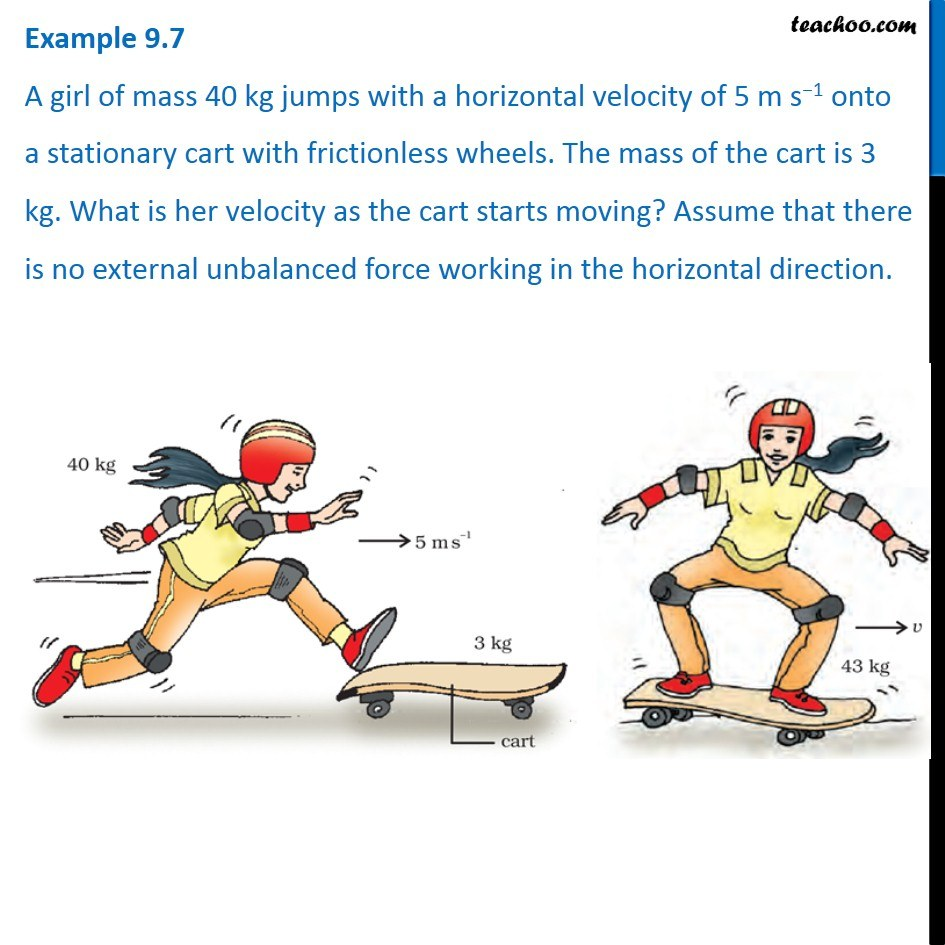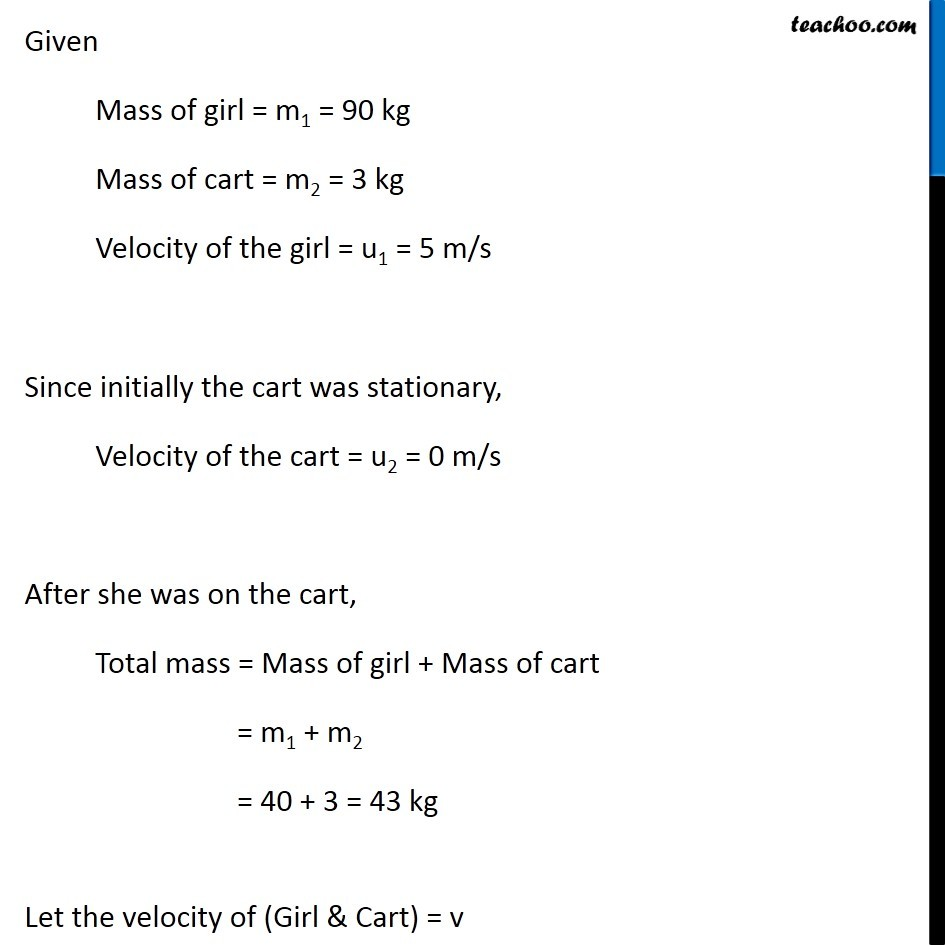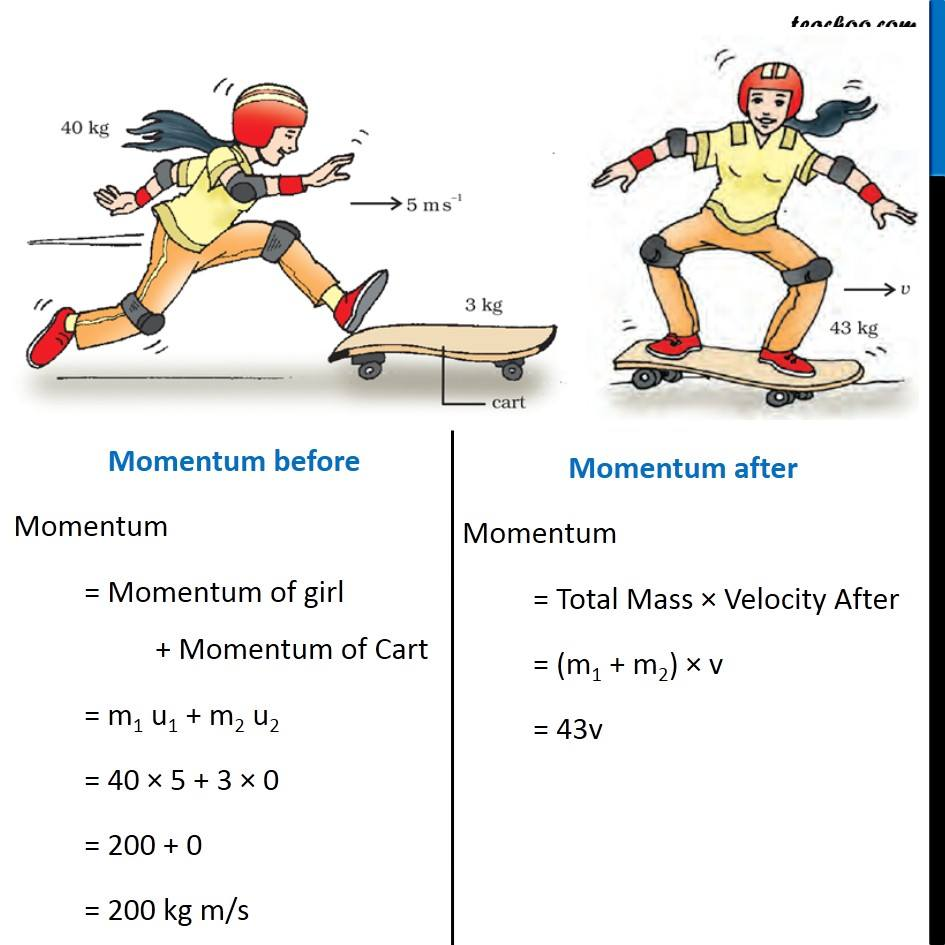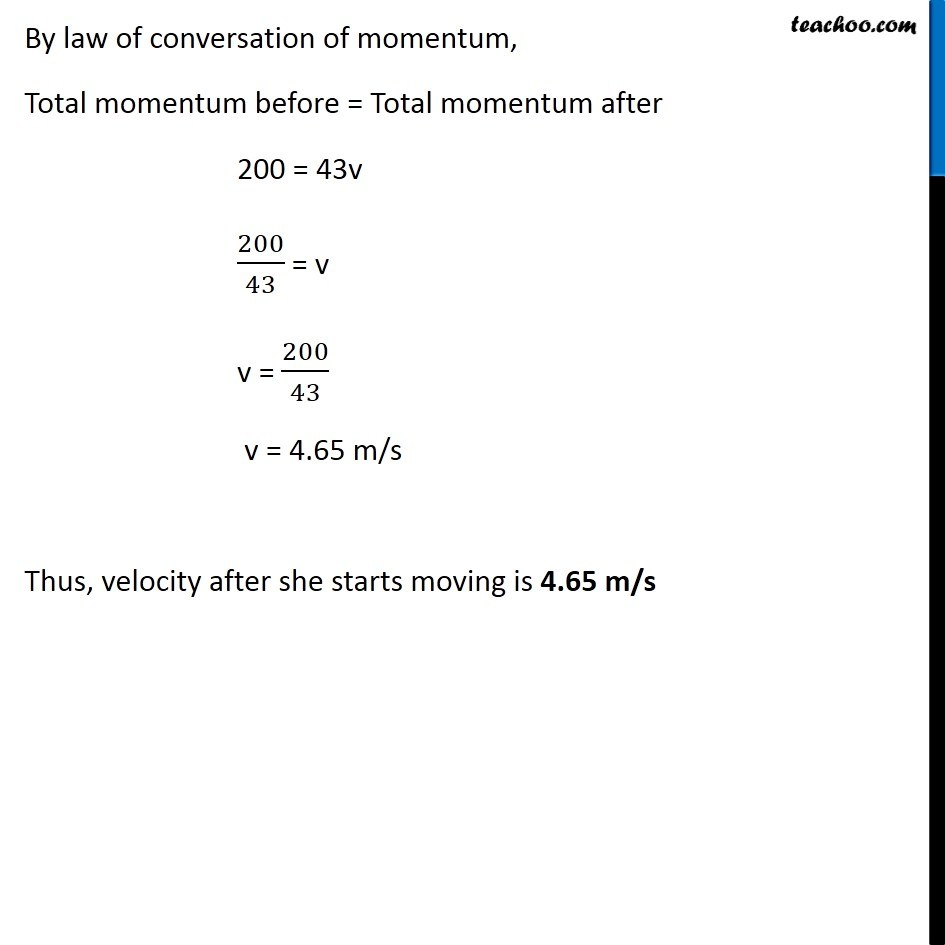Examples from NCERT Book

Class 9
Chapter 9 Class 9 - Force and Laws Of MotionLearn in your speed, with individual attention - Teachoo Maths 1-on-1 Class

### Transcript

Example 9.7 A girl of mass 40 kg jumps with a horizontal velocity of 5 m s−1 onto a stationary cart with frictionless wheels. The mass of the cart is 3 kg. What is her velocity as the cart starts moving? Assume that there is no external unbalanced force working in the horizontal direction. Given Mass of girl = m1 = 90 kg Mass of cart = m2 = 3 kg Velocity of the girl = u1 = 5 m/s Since initially the cart was stationary, Velocity of the cart = u2 = 0 m/s After she was on the cart, Total mass = Mass of girl + Mass of cart = m1 + m2 = 40 + 3 = 43 kg Let the velocity of (Girl & Cart) = v Momentum before Momentum = Momentum of girl + Momentum of Cart = m1 u1 + m2 u2 = 40 × 5 + 3 × 0 = 200 + 0 = 200 kg m/s Momentum after Momentum = Total Mass × Velocity After = (m1 + m2) × v = 43v By law of conversation of momentum, Total momentum before = Total momentum after 200 = 43v 200/43 = v v = 200/43 v = 4.65 m/s Thus, velocity after she starts moving is 4.65 m/s